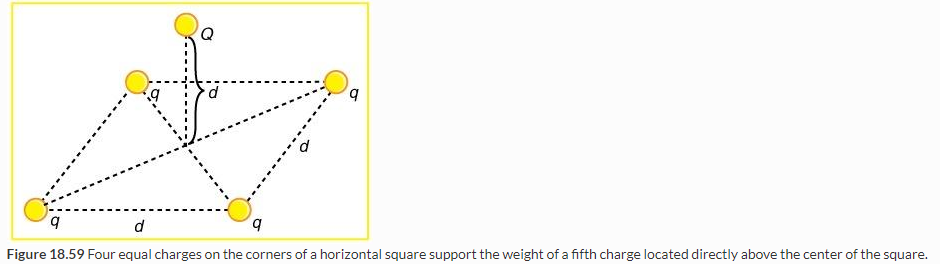# Problem: (a) In Figure 18.59, four equal charges q lie on the corners of a square. A fifth charge Q is on a mass m directly above the center of the square, at a height equal to the length d of one side of the square. Determine the magnitude of q in terms of Q , m , and d , if the Coulomb force is to equal the weight of m. (b) Is this equilibrium stable or unstable? Discuss.

###### FREE Expert Solution

Coulomb's law:

$\overline{){\mathbf{F}}{\mathbf{=}}\frac{\mathbf{k}{\mathbf{q}}_{\mathbf{1}}{\mathbf{q}}_{\mathbf{2}}}{{\mathbf{r}}^{\mathbf{2}}}}$

(a)

A symmetry is created if another charge of magnitude Q is placed directly above the center of the square.

This makes the horizontal components of the forces to cancel leaving the electric force to be equal to the sum of the vertical component of all the forces.

r = sqrt [(half the diagogan)2 + (distance between Q and the center of the square)2)

$\begin{array}{rcl}\mathbf{r}& \mathbf{=}& \sqrt{{\mathbf{\left(}\frac{\mathbf{1}}{\mathbf{2}}\mathbf{d}\sqrt{\mathbf{2}}\mathbf{\right)}}^{\mathbf{2}}\mathbf{+}{\mathbf{d}}^{2}}\\ & \mathbf{=}& \sqrt{\frac{\mathbf{3}}{\mathbf{2}}}\mathbf{d}\end{array}$

Therefore,

$\begin{array}{rcl}\mathbf{F}& \mathbf{=}& \frac{\mathbf{k}\mathbf{q}\mathbf{Q}}{{\mathbf{r}}^{\mathbf{2}}}\\ & \mathbf{=}& \frac{\mathbf{2}}{\mathbf{3}}\frac{\mathbf{q}\mathbf{Q}}{{\mathbf{d}}^{\mathbf{2}}}\\ {\mathbf{F}}_{\mathbf{y}}& \mathbf{=}& \mathbf{F}\mathbf{s}\mathbf{i}\mathbf{n}\mathbf{\theta }\\ \mathbf{s}\mathbf{i}\mathbf{n}\mathbf{\theta }& \mathbf{=}& \frac{\mathbf{d}}{\sqrt{\frac{\mathbf{2}}{\mathbf{3}}}\mathbf{d}}\\ & \mathbf{=}& \sqrt{\frac{\mathbf{2}}{\mathbf{3}}}\end{array}$

The component of force in the y-direction is expressed as:

$\begin{array}{rcl}{\mathbf{F}}_{\mathbf{y}}& \mathbf{=}& \mathbf{4}\mathbf{F}\mathbf{s}\mathbf{i}\mathbf{n}\mathbf{\theta }\\ & \mathbf{=}& \mathbf{4}\mathbf{\left(}\sqrt{\frac{\mathbf{2}}{\mathbf{2}}}\mathbf{\right)}\mathbf{\left(}\frac{\mathbf{2}}{\mathbf{3}}\mathbf{\right)}\mathbf{\left(}\frac{\mathbf{k}\mathbf{q}\mathbf{Q}}{{\mathbf{d}}^{\mathbf{2}}}\mathbf{\right)}\\ & \mathbf{=}& \mathbf{2}\mathbf{.}\mathbf{177}\frac{\mathbf{k}\mathbf{q}\mathbf{Q}}{{\mathbf{d}}^{\mathbf{2}}}\end{array}$

82% (433 ratings)###### Problem Details

(a) In Figure 18.59, four equal charges q lie on the corners of a square. A fifth charge Q is on a mass m directly above the center of the square, at a height equal to the length d of one side of the square. Determine the magnitude of q in terms of Q , m , and d , if the Coulomb force is to equal the weight of m.

(b) Is this equilibrium stable or unstable? Discuss.Frequently Asked Questions

What scientific concept do you need to know in order to solve this problem?

Our tutors have indicated that to solve this problem you will need to apply the Coulomb's Law (Electric Force) concept. You can view video lessons to learn Coulomb's Law (Electric Force). Or if you need more Coulomb's Law (Electric Force) practice, you can also practice Coulomb's Law (Electric Force) practice problems.

What professor is this problem relevant for?

Based on our data, we think this problem is relevant for Professor Premkumar's class at UCSD.